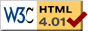### Journée-séminaire de combinatoire

#### (équipe CALIN du LIPN, université Paris-Nord, Villetaneuse)

Le 03 décembre 2015 à 10h00 en B107, Yinon Spinka nous parlera de : Long-range order in random 3-colorings of Z^d

Résumé : Consider a random coloring of a bounded domain in Zd with the probability of each coloring F proportional to exp(-β*N(F)), where β>0 is a parameter (representing the inverse temperature) and N(F) is the number of nea rest neighboring pairs colored by the same color. This is the anti-ferromagnetic 3-state Potts model of statistical physics, used to describe magnetic interactions in a spin system. The Kotecký conjecture is that in such a model, for d≥3 and high enough β, a sampled coloring will typically exhibit long-range order, placing the same color at most of either the even or odd vertices of the domain. We give the first rigorous proof of this fact for large d. This extends previous works of Peled and of Galvin, Kahn, Randall and Sorkin, who treated the case β=infinity. No background in statistical physics will be assumed and all terms will be explained thoroughly. Joint work with Ohad Feldheim.

 Dernière modification : Monday 24 January 2022Contact pour cette page : Cyril.Banderier at lipn.univ-paris13.fr